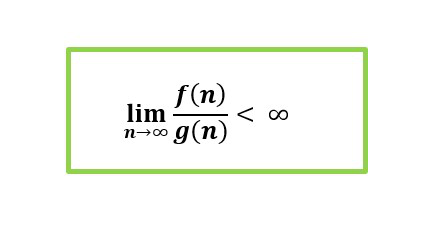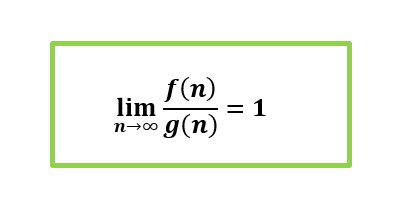Related Articles

# Difference between big O notations and tilde

• Last Updated : 09 Jun, 2021

In asymptotic analysis of algorithms we often encounter terms like Big-Oh, Omega, Theta and Tilde, which describe the performance of an algorithm.
You can refer to the following links to get more insights about asymptotic analysis :

In this article we are going to see the difference between the two notations: Big Oh and Tilde.

1. Big Oh notation (O) :
This notation is basically used to describe the asymptotic upper bound. Mathematically, we can describe it as :

```f(n) = O(g(n))
if there exist positive constants c and
n0 such that
0 <= f(n) <= c*g(n)
for all n >= n0```

The above notation depicts that at the point n=n0, the growth of the function g(n) increases gradually. In algorithms we always deal with larger values of n i.e. n→∞. So, we can also define the Big-Oh notation as :Therefore, f(n) = O(g(n)) if the above limit value lies in the range [ 0 , ∞ )

Example –2. Tilde Notation (~) :
Tilde notation is used when we want to make a simple approximation of a complex function. It simply drops the lower order terms. It is denoted by ~g(n).
If f(n)~g(n) indicates that the value of f(n)/g(n) tends towards 1 for a larger value of n. Mathematically, we can express it as :Example
1.can be written as ~because when we divide both the functions and find the limit for larger value of n, it will become 1.

2 – In an AVL tree before we insert or delete a key, we first compare and find the location of the key. The number of comparisons required in the worst case is :

```h+1, where h is the height of the tree.
The height will be, since AVL tree is a height balanced tree. ```

Therefore, we can replace the value of h in the expression and it becomes :

``

We can ignore the lower order term and it becomes ~. So, the number of comparisons in an AVL tree is approximated to ~.

Some important points about ~ notation in asymptotic analysis –

• It follows equivalence relation property.
• It is identical to big theta notation. There is a minute difference in the arbitrary constant as in big theta notation there can be different values for the constants in the lower bound as well as upper bound but in the case of Tilde, we always get f/g value as 1 or tending towards 1.

The differences between Big Oh and Tilde notations are :

Attention reader! Don’t stop learning now.  Practice GATE exam well before the actual exam with the subject-wise and overall quizzes available in GATE Test Series Course.

Learn all GATE CS concepts with Free Live Classes on our youtube channel.

My Personal Notes arrow_drop_up